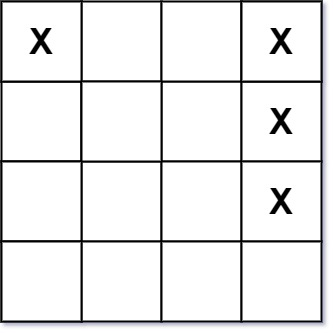# Battleships in a Board

## 1: U-nderstand

Understand what the interviewer is asking for by using test cases and questions about the problem.

• Established a set (2-3) of test cases to verify their own solution later.
• Established a set (1-2) of edge cases to verify their solution handles complexities.
• Have fully understood the problem and have no clarifying questions.
• Have you verified any Time/Space Constraints for this problem?
• Can the input board be blank?
• Let’s assume the board is not blank. We don’t need to consider empty inputs.
• Can battleships be attached to one another?
• There will always be at least one horizontal or vertical cell separating two battleships.
• What is the time and space constraints?
• Time complexity should be `O(M*N)`, m being the rows of the matrix and n being the columns of matrix. Space complexity should be `O(1)`, excluding the recursive stack.
``````HAPPY CASE
Input: board = [["X",".",".","X"],[".",".",".","X"],[".",".",".","X"]]
Output: 2
Explanation: The answer is not 11, because the island must be connected 4-directionally.````````````Input: board = [["."]]
Output: 0

EDGE CASE
Input: board = [["X"]]
Output: 1``````

## 2: M-atch

Match what this problem looks like to known categories of problems, e.g. Linked List or Dynamic Programming, and strategies or patterns in those categories.

For 2D-Array, common solution patterns include:

• Perform a BFS/DFS Search through the 2D Array
• A search through the 2D Array (either BFS or DFS) can help us find each battleship. Which of these two traversals will better help us locate the battleship?
• Hash the 2D Array in some way to help with the Strings
• Hashing would not directly help us find islands. However, we could hash where certain 1's are in the 2D Array to jumpstart searches (BFS/DFS) faster.
• Create/Utilize a Trie
• A Trie would not help us much in this problem since we are not trying to determine anything about a sequence of characters.

## 3: P-lan

Plan the solution with appropriate visualizations and pseudocode.

General Idea: Knowing that a ship can only be horizontal or vertical, we can count the head of ships. A head of ship is where the left side and the up side is ocean or boarder

``````1) Initialize a variable to keep track of the number of battleships
2) Iterate over the board
3) If a 'X' is seen and if the left side and the up side is ocean or at the boarder, then it's the head of a ship and add one to the count.
4) Return number of battleships
``````

⚠️ Common Mistakes

• We can optimize this solution when we reduce the need to follow the route of common solution patterns

## 4: I-mplement

Implement the code to solve the algorithm.

``````class Solution:
def countBattleships(self, board: List[List[str]]) -> int:
# Initialize a variable to keep track of the number of battleships
numberOfBattleships = 0

# Iterate over the board
for i, row in enumerate(board):
for j, cell in enumerate(row):
# If a 'X' is seen and if the left side and the up side is ocean or at the boarder, then it's the head of a ship and add one to the count.
if cell == "X":
if (i == 0 or board[i - 1][j] == ".") and\
(j == 0 or board[i][j - 1] == "."):
numberOfBattleships += 1

# Return number of battleships
return numberOfBattleships``````
``````class Solution {
public int countBattleships(char[][] board) {
if (board == null) {
throw new IllegalArgumentException("Input is null");
}
if (board.length == 0 || board.length == 0) {
return 0;
}

// Initialize a variable to keep track of the number of battleships
int rows = board.length;
int cols = board.length;
int numberOfBattleships = 0;

// Iterate over the board
for (int i = 0; i < rows; i++) {
for (int j = 0; j < cols; j++) {
// If a 'X' is seen and if the left side and the up side is ocean or at the boarder, then it's the head of a ship and add one to the count.
if (board[i][j] == 'X'
&& (j == cols - 1 || board[i][j + 1] == '.')
&& (i == rows - 1 || board[i + 1][j] == '.')) {
numberOfBattleships++;
}
}
}

// Return number of battleships
return numberOfBattleships;
}
}``````

## 5: R-eview

Review the code by running specific example(s) and recording values (watchlist) of your code's variables along the way.

• Trace through your code with an input to check for the expected output
• Catch possible edge cases and off-by-one errors

## 6: E-valuate

Evaluate the performance of your algorithm and state any strong/weak or future potential work.

Assume `N` represents the number of rows in 2D-array. Assume `M` represents the number of columns in 2D-array.

• Time Complexity: `O(N * M)` we need to view each item in the 2D-Array
• Space Complexity: `O(1)`, we only need to store the number of battleships